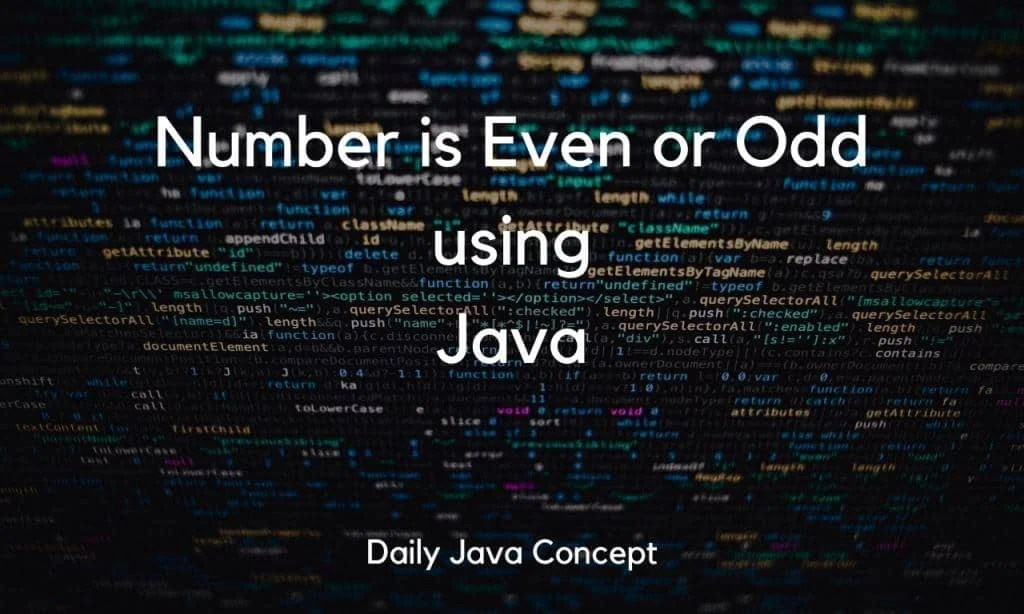# Check Number is Even or Odd without using modulus operator?

0
1021The below program provides the logic of how to check a number is even or odd without using modulus operator. But first, check how to implement it using modulus operator.

### Using Modulus Operator :

```import java.util.Scanner;

public class EvenOdd {

public static void main(String[] args) {

// Scanner class use to take the user Input.
Scanner sc = new Scanner(System.in);

System.out.println("Enter the Number : " );

// Variable n store the User input
int n = sc.nextInt();

// If Number is divided by 2 and leave a remainder 0 then
// that number is an Even Number otherwise Number is Odd number;

// Using modulus Operator it returns the remainder and then we compare the remainder with 0.

if(n%2==0){
System.out.println(String.format("Number %d is Even Number.",n));
}else{
System.out.println(String.format("Number %d is Odd Number.",n));
}
}
}
```

Output 1:

```Enter the Number :
74
Number 74 is Even Number.```

Output 2:

```Enter the Number :
91
Number 91 is Odd Number.```

But this Simple Question some times becomes tricky when it is asked in an interview. In the Interview, the question is like “Check a number is even or odd without using modulus Operator.”

so there are 2 ways to solve this question. Try both methods to solve and find the best one for you.

### Using Division Method :

```import java.util.Scanner;

public class EvenOdd {

public static void main(String[] args) {

// Scanner class use to take the user Input.
Scanner sc = new Scanner(System.in);

System.out.println("Enter the Number : " );

// Variable n store the User input
int n = sc.nextInt();

// If Number is divided by 2 and leave a remainder 0 then
// that number is an Even Number otherwise Number is Odd number;

//Using Division method we first divide the number by 2 and the multiply it with 2 and check,
// if both number equal the number is even otherwise number is odd

int quotient = n/2;

if(quotient*2 == n){
System.out.println(String.format("Number %d is Even Number.",n));
}else{
System.out.println(String.format("Number %d is Odd Number.",n));
}
}
}
```

Output 1:

```Enter the Number :
74
Number 74 is Even Number.```

Output 2:

```Enter the Number :
91
Number 91 is Odd Number.```

In the Division method we first divide a number by 2 then we multiply it by 2 and compare both numbers is equal or not?

If both numbers are equal then the number is Even otherwise the number is Odd.

Example:  Here given an example, to make you understand this method of solving the problem:

User Input: 73

Step 1: Divide number by 2 gives 36 and remainder as 1 but in this case, the remainder is omitted because we are not using it.

Step 2: Then multiply the number by 2 gives 72.

Step 3: Now, compare the User input and the number we got after multiplying. In this case, both numbers are not the same so we simply said that the User Input is an Odd number.

For Confirmation, you can check this number with the Modulus Method.

### Using Bitwise AND Operator :

```import java.util.Scanner;

public class EvenOdd {

public static void main(String[] args) {

// Scanner class use to take the user Input.
Scanner sc = new Scanner(System.in);

System.out.println("Enter the Number : " );

// Variable n store the User input
int n = sc.nextInt();

// If Number is divided by 2 and leave a remainder 0 then
// that the number is an Even Number otherwise Number is Odd number;

// Using Bitwise AND Operator, the numbers are in the form of 2's Compliment
// and the LSB(Least Significant Bit) is 0, so we check if LSB is 0 then Number is Even,
// otherwise Number is Odd.

if((n & 1) == 0){
System.out.println(String.format("Number %d is Even Number.",n));
}else{
System.out.println(String.format("Number %d is Odd Number.",n));
}
}
}
```

Output 1:

```Enter the Number :
74
Number 74 is Even Number.```

Output 2:

```Enter the Number :
91
Number 91 is Odd Number.```

In this method, we first apply the Bitwise AND Operator between the user input and 1 that gives the output as 0 or 1 and then compare it with 0,

if equals to 0 then number are even otherwise the number is Odd.

Example:  Here given an example, to make you understand this method of solving the problem:

User Input: 74

Step 1: First we apply the Bitwise AND operator between user input and 1.

the Binay representation of 74 is 1001000 and Binary representation of 1 is 0000001.

Bitwise AND operator return 1 if the bits at corresponding position is 1 otherwise 0.

74 —–>       1001000

1 ——>        0000001

output ->      0000000  ——–> 0

No bit at their corresponding position is 1 so the output is 0.

Step 3: Now, compare the output we got after applying Bitwise AND operator with 0, here output matches the condition and returns 74 is and Even Number.

For Confirmation, you can check this number with the Modulus Method.

Hope you like this post.